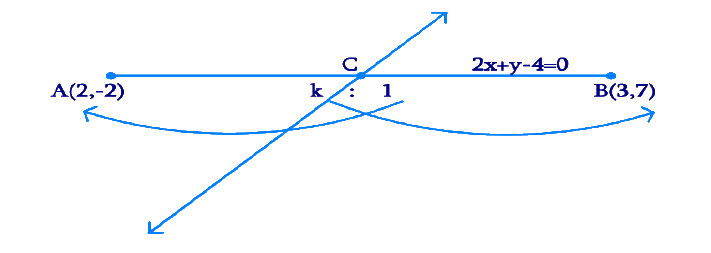# Ex.7.4 Q1 Coordinate Geometry Solution - NCERT Maths Class 10

Go back to  'Ex.7.4'

## Question

Determine the ratio in which the line\begin{align}2x + y - {\text{ }}4{\text{ }} = {\text{ }}0\end{align} divides the line segment joining the points \begin{align} A\left( {2,\,\, - {\text{ }}2} \right){\text{ and }}{\text{ }}B \left( {3,\,\,7} \right)\end{align}.

## Text Solution

Reasoning:

The coordinates of the point$$\;{P}\,(x, y)$$ which divides the line segment joining the points $${A(x1, y1) \;\text{and}\; B(x_2, y_2)}$$, internally, in the ratio $$\rm{m1\,:\,m2}$$ is given by the Section Formula.

\begin{align}{{P}}({\text{x}},{\text{y}}) = \left[ {\frac{{{\text{m}}{{\text{x}}_2} + {\text{n}}{{\text{x}}_1}}}{{{\text{m}} + {\text{n}}}},\frac{{{\text{m}}{{\text{y}}_2} + {\text{n}}{{\text{y}}_1}}}{{{\text{m}} + {\text{n}}}}} \right] & & \end{align}

What is known?

The $$x$$ and $$y$$ co-ordinates of the points $$A$$ and $$B$$.

The equation of the straight line i.e. \begin{align}2x + y - {\text{ }}4{\text{ }} = {\text{ }}0\end{align}

What is unknown?

The ratio in which the line \begin{align}2x + y - {\text{ }}4{\text{ }} = {\text{ }}0\end{align} divides the line segment joining the points \begin{align}{\text{ A}}\left( {2,\,\, - {\text{ }}2} \right){\text{ }}{\text{ and}}{\text{ B}}\left( {3,\,\,7} \right)\end{align}

Steps:

From the Figure,Given,

• Let the given line \begin{align}2x + y - 4{\text{ }} = 0\end{align} divide the line segment joining the points \begin{align}A\left( {2,{\text{ }} - 2} \right){\text{ }}{\text{and }}B\left( {3,{\text{ }}7} \right)\end{align} in a ratio $$k : 1$$ at point $$C$$.

Coordinates of the point of division, \begin{align}\text{C}(x,y) = \left( {\frac{{3k + 2}}{{k + 1}},\,\,\frac{{7k - 2}}{{k + 1}}} \right)\end{align}

This point $$C$$ also lies on \begin{align}2x + y - {\text{ }}4{\text{ }} = {\text{ }}0 & & \ldots \ldots \ldots {\text{Equation }}\left( 1 \right)\end{align}

By substituting the values of \begin{align}\text{C}\left( {x,{\text{ }}y} \right)\end{align} in Equation (1),

\begin{align} ∴\; 2\left( {\frac{{3k + 2}}{{k + 1}}} \right) + \left( {\frac{{7k - 2}}{{k + 1}}} \right) - 4 = 0 \end{align}

\begin{align} \frac{{6k + 4 + 7k - 2 - 4k - 4}}{{k + 1}} = 0 \qquad \quad \end{align} (By Cross multiplying & transposing)

\qquad\qquad\begin{align} 9k - 2 = 0 \end{align}

\qquad\qquad\begin{align}k = \frac{2}{9}\end{align}

Therefore, the ratio in which the line \begin{align}2x + y - {\text{ }}4{\text{ }} = {\text{ }}0\end{align} divides the line segment joining the points \begin{align}{{A }}\left( {2,{\text{ }} - 2} \right){\text{ }}{\text{ and}}\;{{B }}\left( {3,{\text{ }}7} \right)\end{align} is $$2:9$$ internally.

Learn from the best math teachers and top your exams

• Live one on one classroom and doubt clearing
• Practice worksheets in and after class for conceptual clarity
• Personalized curriculum to keep up with school